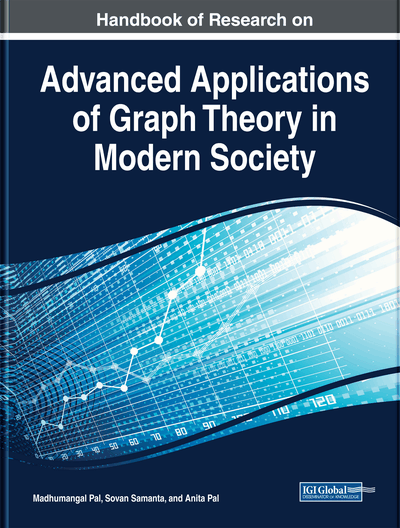# The Hyper-Zagreb Index and Some Properties of Graphs

Rao Li (University of South Carolina at Aiken, USA)
DOI: 10.4018/978-1-5225-9380-5.ch006
Available
\$37.50
No Current Special Offers

## Abstract

Let G = (V(G), E(G)) be a graph. The complement of G is denoted by Gc. The forgotten topological index of G, denoted F(G), is defined as the sum of the cubes of the degrees of all the vertices in G. The second Zagreb index of G, denoted M2(G), is defined as the sum of the products of the degrees of pairs of adjacent vertices in G. A graph Gisk-Hamiltonian if for all X ⊂V(G) with|X| ≤ k, the subgraph induced byV(G) - Xis Hamiltonian. Clearly, G is 0-Hamiltonian if and only if G is Hamiltonian. A graph Gisk-path-coverableifV(G) can be covered bykor fewer vertex-disjoint paths. Using F(Gc) and M2(Gc), Li obtained several sufficient conditions for Hamiltonian and traceable graphs (Rao Li, Topological Indexes and Some Hamiltonian Properties of Graphs). In this chapter, the author presents sufficient conditions based upon F(Gc) and M2(Gc)for k-Hamiltonian, k-edge-Hamiltonian, k-path-coverable, k-connected, and k-edge-connected graphs.
Chapter Preview
Top

## Introduction

We consider only finite undirected graphs without loops or multiple edges. Notation and terminology not defined here follow that in Bondy and Murty (1976). Letbe a graph. We use n, e, δ, and κ to denote the order, size, minimum degree, and connectivity of G, respectively. The complement of G is denoted by. We also useandto denote the complete graph and the empty graph of order n. The forgotten topological index of G, denoted, is defined as(see Furtala & Gutman, 2015). The second Zagreb index of G, denoted, is defined as(see Gutman et al., 1975). The hyper-Zagreb index of G, denoted, is defined as(see Milovanovic, Matejic & Milovanovic, 2019). We useto denote the largest eigenvalue of the adjacency matrix of a graph G of order n. For two disjoint graphsand, we useandto denote respectively the union and join ofand. The concept of closure of a graph G was introduced by Bondy and Chvátal in Bondy and Chvatal (1976). The k-closure of a graph G, denoted, is a graph obtained from G by recursively joining two nonadjacent vertices such that their degree sum is at least k until no such pair remains. We useto denote the number of r-combinations of a set with n distinct elements. A cycle C in a graph G is called a Hamiltonian cycle of G if C contains all the vertices of G. A graph G is called Hamiltonian if G has a Hamiltonian cycle. A path P in a graph G is called a Hamiltonian path of G if P contains all the vertices of G. A graph G is called traceable if G has a Hamiltonian path. A graph G is k-Hamiltonian if for allwith, the subgraph induced byis Hamiltonian. Clearly, G is 0-Hamiltonian if and only if G is Hamiltonian. A graph G is k-edge-Hamiltonian if any collection of vertex-disjoint paths with at most k edges is in a Hamiltonian cycle in G. Clearly, G is 0-edge-Hamiltonian if and only if G is Hamiltonian. A graph G is k-path-coverable ifcan be covered by k or fewer vertex-disjoint paths. Clearly, G is 1-path-coverable if and only G is a traceable. A graph G is k-connected if it has more than k vertices and G is still connected whenever fewer than k vertices are removed from G. A graph G is k-edge-connected if it has at least two vertices and G is still connected whenever fewer than k edges are removed from G.

## Complete Chapter List

Search this Book:
Reset